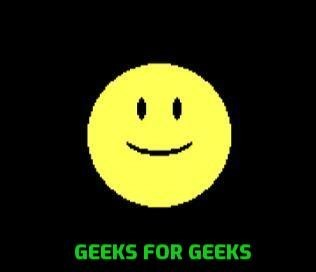# Draw a smiley face using Graphics in C language

• Last Updated : 19 Apr, 2021

Prerequisite: graphics.h, How to include graphics.h in CodeBlocks?
The task is to write a C program to draw a smiley face using graphics in C.
To run the program we have the include the below header file:

`#include <graphic.h>`

Approach: We will create a Smiley Face with the help below functions:

1. fillellipse(int x, int y, int x_radius, int y_radius): A function from graphics.h header file which draws and fills an ellipse with center at (x, y) and (x_radius, y_radius) as x and y radius of ellipse.
2. ellipse(int x, int y, int stangle, int endangle, int xradius, int yradius): A function from graphics.h header file which is used to draw an ellipse (x, y) are coordinates of the center of the ellipse, stangle is the starting angle, end angle is the ending angle, and fifth and sixth parameters specifies the X and Y radius of the ellipse.
3. setcolor(n): A function from graphics.h header file which set the color of pointer(cursor).
4. setfillstyle(): A function from graphics.h header file which sets the current fill pattern and fill color.
5. floodfill(): A function from graphics.h header file which is used to fill an enclosed area.

Below is the implementation of to draw Smiley Face using graphics in C:

## C

 `// C program to create a smiley face``#include ``#include ``#include ``#include ` `// Driver Code``int` `main()``{` `    ``// Initialize graphic driver``    ``int` `gr = DETECT, gm;` `    ``// Initialize graphics mode by passing``    ``// three arguments to initgraph function` `    ``// &gdriver is the address of gdriver``    ``// variable, &gmode is the address of``    ``// gmode and  "C:\\Turboc3\\BGI" is the``    ``// directory path where BGI files``    ``// are stored``    ``initgraph(&gr, &gm, ``"C:\\Turboc3\\BGI"``);` `    ``// Set color of smiley to yellow``    ``setcolor(YELLOW);` `    ``// creating circle and fill it with``    ``// yellow color using floodfill.``    ``circle(300, 100, 40);``    ``setfillstyle(SOLID_FILL, YELLOW);``    ``floodfill(300, 100, YELLOW);` `    ``// Set color of background to black``    ``setcolor(BLACK);``    ``setfillstyle(SOLID_FILL, BLACK);` `    ``// Use fill ellipse for creating eyes``    ``fillellipse(310, 85, 2, 6);``    ``fillellipse(290, 85, 2, 6);` `    ``// Use ellipse for creating mouth``    ``ellipse(300, 100, 205, 335, 20, 9);``    ``ellipse(300, 100, 205, 335, 20, 10);``    ``ellipse(300, 100, 205, 335, 20, 11);` `    ``getch();` `    ``// closegraph function closes the``    ``// graphics mode and deallocates``    ``// all memory allocated by``    ``// graphics system``    ``closegraph();` `    ``return` `0;``}`

Output:
Below is the output of the above program:My Personal Notes arrow_drop_up